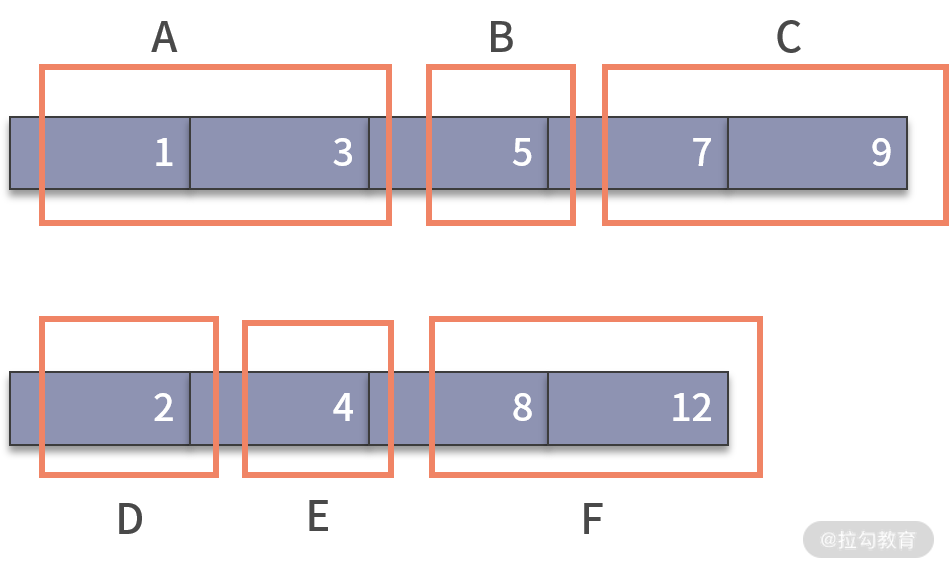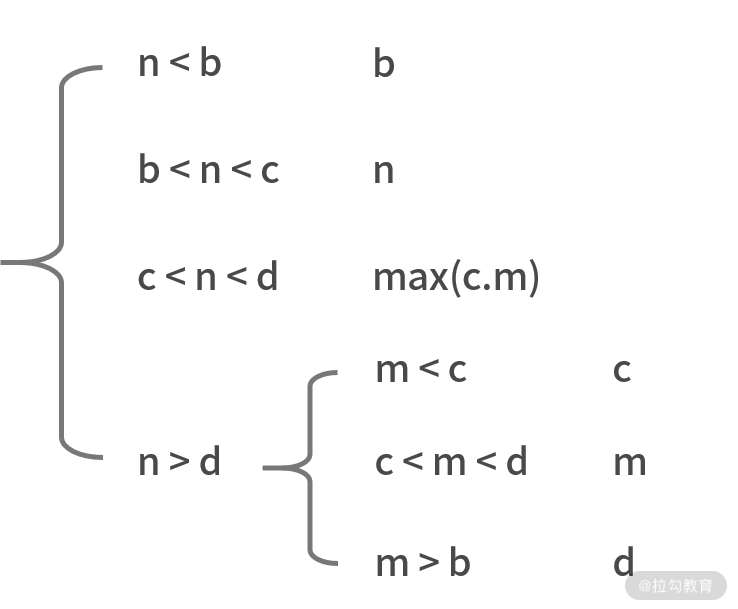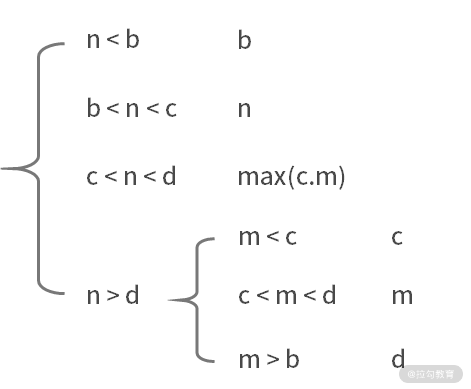18 真题案例（三）：力扣真题训练

力扣真题训练

1. 复杂度分析。估算问题中复杂度的上限和下限。
2. 定位问题。根据问题类型，确定采用何种算法思维。
3. 数据操作分析。根据增、删、查和数据顺序关系去选择合适的数据结构，利用空间换取时间。
4. 编码实现

例题 1：删除排序数组中的重复项

【题目】 给定一个排序数组，你需要在原地删除重复出现的元素，使得每个元素只出现一次，返回移除后的数组和新的长度，你不需要考虑数组中超出新长度后面的元素。

【解析】 这个题目比较简单，应该是送分题。不过，面试过程中的送分题也是送命题。这是因为，如果送分题没有拿下，就会显得非常说不过去。public static void main(String[] args) {
int[] nums = {0,0,1,1,1,2,2,3,3,4};
int temp = nums;
int len = 1;
for (int i = 1; i < nums.length; i++) {
if (nums[i] != temp) {
nums[len] = nums[i];
temp = nums[i];
len++;
}
}
System.out.println(len);
for (int i = 0; i < len; i++) {
System.out.println(nums[i]);
}
}

例题 2：查找两个有序数组合并后的中位数

【题目】 两个有序数组查找合并之后的中位数。给定两个大小为 m 和 n 的正序（从小到大）数组 nums1 和 nums2。请你找出这两个正序数组合在一起之后的中位数，并且要求算法的时间复杂度为 O(log(m + n))。

nums2 = [2, 4, 8, 12]

【解析】 这个题目是我个人非常喜欢的，原因是，它所有的解法和思路，都隐含在了题目的描述中。如果你具备很强的分析和解决问题的能力，那么一定可以找到最优解法。1. 如果 m < b，则结果为 b；
2. 如果 b < m < c，则结果为 m；
3. 如果 m > c，则结果为 c。

1. 如果 m < c，则结果为 c；
2. 如果 c < m < d，则结果为 m；
3. 如果m > d，则结果为 d。

1. 如果 n < b，则结果为 b；
2. 如果 b < n < c，则结果为 n；
3. 如果 c < n < d，则结果为 max(c,m)；
4. 如果 n > d，m < c，则结果为 c；
5. 如果 n > d，c < m < d，则结果为 m；
6. 如果 n > d，m > d，则结果为 d。1. 如果 n < b，则结果为 b；
2. 如果 b < n < c，则结果为 n；
3. 如果 c < n < d，则结果为 max(c,m)；
4. 如果 n > d，m < c，则结果为 c；
5. 如果 n > d，c < m < d，则结果为 m；
6. 如果 n > d，m > d，则结果为 d。与可能性 3 完全一致。

public static void main(String[] args) {
int[] nums1 = {1,2,3,4,5};
int[] nums2 = {6,7,8};
int median = getMedian(nums1,0, nums1.length-1, nums2, 0, nums2.length-1);
System.out.println(median);
}

public static int getMedian(int[] a, int begina, int enda, int[] b, int beginb, int endb ) {
if (enda - begina == 0) {
return a[begina] > b[beginb] ? b[beginb] : a[begina];
}
if (enda - begina == 1){
if (a[begina] < b[beginb]) {
return b[beginb] > a[enda] ? a[enda] : b[beginb];
}
else {
return a[begina] < b[endb] ? a[begina] : b[endb];
}
}
if (endb-beginb < 2) {
if ((endb - beginb == 0) && (enda - begina)%2 == 0) {
int m = b[beginb];
int bb = a[(enda + begina)/2 - 1];
int c = a[(enda + begina)/2];
return (m < bb) ? bb : (m < c ? m : c);
}
else if ((endb - beginb == 0) && (enda - begina)%2 != 0) {
int m = b[beginb];
int c = a[(enda + begina)/2];
int d = a[(enda + begina)/2 + 1];
return m < c ? c : (m < d ? m : d);
}
else {
int m = b[beginb];
int n = b[endb];
int bb = a[(enda + begina)/2 - 1];
int c = a[(enda + begina)/2];
int d = a[(enda + begina)/2 + 1];
if (n<bb) {
return bb;
}
else if (n>bb && n < c) {
return n;
}
else if (n > c && n < d) {
return m > c ? m : c;
}
else {
return m < c ? c : (m<d ? m : d);
}
}
}
else {
int mida = (enda + begina)/2;
int midb = (endb + beginb)/2;
if (a[mida] < b[midb]) {
int step = endb - midb;
return getMedian(a,begina + step, enda, b, beginb, endb - step);
}
else {
int step = midb - beginb;
return getMedian(a,begina,enda - step, b, beginb+ step, endb );
}
}